Reach Us+32-10-28-02-25
On De Broglies Double-particle Photon Hypothesis | OMICS International
Journal of Physical Mathematics
All submissions of the EM system will be redirected to Online Manuscript Submission System. Authors are requested to submit articles directly to Online Manuscript Submission System of respective journal.

On De Broglies Double-particle Photon Hypothesis

André Michaud*

*Corresponding Author:
André Michaud
Tel:
+1 418 624 0608
E-mail: [email protected]

Received Date: October 17, 2015; Accepted Date: December 11, 2015; Published Date: January 16, 2016

Citation: Michaud A (2016) On De Broglie’s Double-particle Photon Hypothesis. J Phys Math 7:153. doi:10.4172/2090-0902.1000153

Copyright: © 2016 Michaud A. This is an open-access article distributed under the terms of the Creative Commons Attribution License, which permits unrestricted use, distribution, and reproduction in any medium, provided the original author and source are credited.

Visit for more related articles at Journal of Physical Mathematics

Abstract

Establishment of an LC equation and of a local fields equation describing permanently localized photons from the analysis of kinetic energy circulation within the energy structure of the double-particle photon that Louis de Broglie hypothesized in the early 1930's. Among other interesting features, these equations provide a mechanical explanation to the localized photon properties of self-propelling at the speed of light and of self-guiding in straight line when no external interaction tends to deflect its trajectory. This paper summarizes the seminal considerations that led to the development of the 3-spaces model.

Keywords

Electromagnetic theory; Kinetic energy; Photon; Acceleration; Electron-positron pairs; 1.022 MeV LC equation; 3-spaces

Introduction

The first integrated representation of electromagnetic energy was provided by Maxwell as a continuous wave phenomenon that would be due to interacting electric and magnetic fields inducing each other, which led to the recognition that radio frequencies belong to the same electromagnetic spectrum as visible light. Then came Planck's analysis of Wien’s experimental data on the black body demonstrating that electromagnetic energy is always captured as frequency dependent discrete amounts. Einstein’s photoelectric proof confirmed Planck's hypothesis shortly afterwards by demonstrating that photons do behave as if they were separate localized quanta when intercepted while also demonstrating that they possess longitudinal inertia, which eventually earned them both Nobel prizes. Compton and Raman added further experimental confirmation of Planck’s conclusion, while experimenting with other types of collisions between photons and electrons. These findings conclusively confirmed the discrete and point-like behavior of photons when being absorbed. We must also keep in mind that an interaction cross-section always larger than zero needs to be assumed for all point-like behaving particles during scattering experiments to correctly account for the observed recorded traces. So we know that photons are not really point-like with zero dimensions in the mathematical sense, even if their motion can be calculated as if they were; just like the trajectory of the Moon about the Earth is calculated as if their masses were concentrated in a single point at the center of each body. Point-like behavior of photons upon emission was also subsequently understood and verified, which we will have a look at further on. So, we know for certain that Maxwell's continuous "waves" do not exist as such at the submicroscopic level, despite the fact that his equations allow calculating all electromagnetic manifestations with the utmost precision when electromagnetic energy is treated as being continuous and featureless as observed from our macroscopic perspective.

In fact, what these discoveries reveal is that we are in the very same situation with respect to electromagnetic energy that we are in with respect to solid materials, as it closely parallels the fact that although we can observe that the surface of a polished diamond has a flawlessly smooth finish from our macroscopic perspective, for example, we can also alternately observe that this same surface is granular and bumpy when the scattering particles of an electron microscope reveal the outlines of the individual atoms making up the crystal surface at the submicroscopic level. In the latter case however, we have a rather extensive understanding of the inner structure of the atoms involved, but to this date, the inner structure of photons is still the object of speculation.

For the past century, there has been a deeply ingrained conception in the case of light that it sometimes behaves as a wave and sometimes as a particle, two types of behavior that are incompatible for a number of reasons and that gave rise to the concept of "wave-particle behavior" to characterize the photon. Close examination of the concept in light of the macroscopic-submicroscopic comparison just clarified leads to the view that generally speaking, "wave behavior" could simply be the result of behavior of crowds of discrete photons that our macroscopic instruments generally deal with while "particle behavior" could simply be the behavior of individual photons at the submicroscopic level. This would go a long way in removing the inherent incompatibility of the "wave-particle behavior" concept, by replacing it with a "macroscopic-wave behavior vs submicroscopic particle behavior" concept. But we will see further on that with the model that will be proposed here, even at the submicroscopic level, the localized photon can display both types of behavior without any conflict by associating transverse wave behavior with longitudinal particle behavior. Also, despite its systematic point-like behavior in all scattering and capture experiments, behavior typical of elementary particles, the photon was suspected early on of not being elementary because light can be polarized, which cannot be explained if the photon was made up of a single point-like behaving particle. This was clarified by Louis de Broglie as the concept of spin was introduced, associating a spin of 1/2 to point-like behaving particles that were proven out of any doubt to be really elementary, such as the electron and the positron, and consequently a spin of 1 to the photon, thus hypothesizing that if it was made up of two particles, this could directly explain why light can be polarized .

Louis de Broglie was the first to elaborate a comprehensive theory on the possible internal structure of photons. According to his hypothesis as proposed in the 1930's, a permanently localized photon following a least action trajectory can satisfy at the same time Bose-Einstein's statistic and Planck's Law, perfectly explain the photoelectric effect while obeying Maxwell's equations and totally conform to the properties of Dirac’s theory of complementary corpuscles symmetry, only if it involves two particles, or half-photons of spin 1/2, " that must be complementary with respect to each other in the same manner that the positive electron (the positron) is complementary to the negative electron in the Dirac Hole Theory" .

The following other quotes from the same reference summarize his hypothesis:

"Such a complementary couple of particles is likely to annihilate at the contact of matter by relinquishing all of its energy, which perfectly accounts for the characteristics of the photoelectric effect."

Furthermore:

"The photon, being made up of two elementary particles of spin h/4π, will obey the Bose-Einstein statistic as required by the precision of Planck's law for the black body."

Finally, he concludes that:

"this model of the photon allows the definition of an electromagnetic field linked to the probability of annihilation of the photon, a field that obeys Maxwell's equations and has all the characteristics of electromagnetic light waves."

Over the course of the 1930's and 1940's, de Broglie and his students progressively came up with an interesting and workable solution based on wave mechanics, that involved both corpuscles being singularities in an underlying wave phenomenon . After Quantum Chromodynamics was developed in the 1970's an alternate model was developed, involving a mix of quark-antiquark pairs and gluons  based on this new theory and Quantum Mechanics, which also is an interesting and workable approach. A few other models have been proposed since, but all approaches have the similar downside with respect to Maxwell’s theory of treating the electric and magnetic fields, either explicitly or implicitly, as a single "electromagnetic field" which turns out to be somehow featureless at the general level (the electromagnetic tensor), which distracts from permanent awareness that both fields are of equal and separate importance in Maxwell's theory, with different and irreconcilable characteristics, besides mutually inducing each other. This left no precise function being assigned to the "magnetic" aspect of the electromagnetic energy in a possible mechanics of mutual induction that would also involve the two separate charges, which are the "electric" components of the photon, a mechanics that would explain why photons can maintain sufficient local unity to account for their systematic and verifiable point-like behavior during scattering or absorption encounters, which includes all photons that we know have been emitted from the farthest reaches detectable in the universe, after having traveled for countless years. Indeed, the twin "electric" particles end up in both models as having an existence separate from the electric aspect of the electromagnetic energy that the localized photon is meant to represent, which introduces the required twin particles in a manner that does not incorporate them into the sequence of the electric vs magnetic mutual induction cycle that they theoretically are meant to enhance, according to de Broglie's initial hypothesis:

"it seemed to me that to obtain a clear image, in agreement with the classical concepts of the wave-particle dualism with respect to space and time, it was required to succeed in incorporating the particle into the wave" .

But it seems that the non-deterministic trend that was prevailing after the 1927 Solvey Congress confronted him with such difficulties that he ended up renouncing this ultimate goal . Generalizing the electromagnetic interaction as a single tensor is a fine approach to obtain global perspectives, but it seems that looking for ever more detailed descriptions always favored deeper understanding of physical issues. This paper is then an attempt at exploring deeper even than the already interestingly detailed electric and magnetic fields as described by Maxwell's theory.

The Required Internal Electromagnetic Symmetry

As it stands, if the photon's double-component electric aspect is to remain coherent with its point-like behavior at the moments of emission and capture (or scattering), however long the time elapsed and distance covered between both events, the two separate "electric" half-photons have to unite in some fashion during each cycle of the photon’s frequency to maintain point-like localization and most importantly to incorporate into the mechanical process the other half of the electromagnetic relation, that is, its magnetic aspect. Doesn't the induction of an increasing magnetic field inseparable from changing current due to moving charges immediately come to mind at this point? In the case of photons, this brings displacement current into the picture, which would involve local motion of the postulated double charges that would cause the required change in the local electric field within the photon quantum, a current that would come into being without the presence of matter in this case, a process that interestingly was first proposed by Maxwell himself in 1865 and was the foundation of his electromagnetic theory  This in turn hints at the possibility of an internal oscillation of the photon energy related to its frequency.

Let’s keep in mind here that the term “frequency” applies to any sort of cyclic motion, be it rotational, translational on a closed orbit or any other type of oscillatory motion, from simple sinusoidal harmonic motion to the cyclic translational reciprocating "swing" between two states being considered here and that we will term “oscillation” for simplicity’s sake. This means that all aspects of angular momentum that we naturally associate with rotary motion can also be applied to reciprocating motion, which in turn allows the "spin" of elementary particles to be hypothesized as possibly corresponding to a reciprocating motion of the energy concerned without changing in any way the equations that already account for it.

It is a fact that all experimental research aimed at identifying charges in electromagnetic waves have failed to detect any in support of Maxwell's assumption. But let’s consider that if electromagnetic waves as Maxwell conceived them really turn out to be only a convenient mathematical representation of a macroscopic perception of a crowd effect due to the presence of countless localized moving photons at the submicroscopic level, it would indeed be these individual photons that would display the searched for charges and would be the local sites of displacement current versus magnetic induction activity.

However, there exists no instrument sensitive enough to detect the infinitesimal fields of individual photons, with the added difficulties that they move at the speed of light and that any interception of a single photon simply incorporates its energy as an infinitesimal kinetic energy increment to one electron in one atom of the material that the detector is made of. But since this postulate was such a major and fruitful foundation in the elaboration of Maxwell's theory, which in turn allows such precise calculations, there seems to be no reason to do away with it now. The double-particle photon hypothesis would then imply that photons have to be stable localized moving electromagnetic structures whose energy quantum could logically only alternate between a two components electric state, with both components separating in space (an electric dipole), and a magnetic state involving only one component to explain permanent localization and that could consequently be dipolar in only one manner. Total symmetry of the magnetic aspect involving a single component can be obtained only if it consists in a single spherically expanding phase as both electric components move towards each other, followed by a spherical contraction phase as both electric components move away from each other; both magnetic expansion and contraction sequences of the magnetic component being normal to the electric phase at all times. This also means that the single magnetic component of the photon can be dipolar only along the time dimension since both expansion and contraction sequences cannot possibly occur simultaneously. Such a dynamic structure would still preserve the required fundamental symmetry since the spacewise moving electric dipole would be permanently counterbalanced by a related time-wise perpendicularly moving magnetic dipole, with both dipoles remaining perpendicular to the direction of motion of the photon in space, thus obeying the triple orthogonality required for plane wave treatment in Maxwell's theory's for straight line motion of electromagnetic energy.

Electrostatically Destabilizing Trajectories Intersections

It must be considered also that Quantum Electrodynamics implicitly recognizes the presence of Coulomb interaction between a decoupling photon and a heavy nucleus, since it incorporates a Feynman's "virtual photon" into the pair production process representation (Figure 1), which was explicitly defined by Feynman himself as being a metaphor for Coulomb interaction , thus indirectly recognizing that Coulomb interaction has to be in action between the photon and the destabilizing heavy particle even before the pair separates, whatever the sign status of the photon's internal charges may have been. Let's consider what is likely to occur when a photon of energy 1.022 MeV or more grazes very closely a heavy atomic nucleus. We know since de Broglie that all massive and charged elementary particles are electromagnetic in nature, since electric charges cannot be dissociated from a magnetic counterpart. This includes of course the scatterable point-like behaving massive up and down quarks making up the inner scatterable structure of nucleons (protons and neutrons) since they also possess measurable electric charges, charged quarks whose existence was not yet known when de Broglie was actively working on his hypothesis, since they were experimentally scattered against only in the late 1960's . Destabilization leading to pair decoupling could then be explainable by the presence of these point-like behaving electromagnetic charged elementary particles of which all nucleons making up atomic nuclei are made, that can presumably enter into homo- and/or heterostatic interaction with the charges of the half-photons while the photon is in its electrostatic phase as it flies by. It becomes just as obvious then that these interactions may then become more and more intense in relation with the inverse square of the diminishing distance that separates the half-photons from these up and down quarks if a Coulomb-like law effectively applies, a process represented in Quantum Electrodynamics by the Feynman diagram shown in Figure 1 . The fact that such decoupling can occur only during moments of very close proximity between photon and nucleus comes in support of the presence of an interaction as a function of the inverse square of the distance such as the Coulomb law. Similarly, pair creation during close flyby of two photons, at least one of which exceeding the 1.022 MeV minimum energy threshold without any atomic nuclei being close by, such as was first experimentally confirmed by Kirk McDonald et al. at the Stanford Linear Accelerator in 1997 with experiment #e144 , is represented by the Feynman diagram shown in Figure 2 . So there seems to exist sufficient supporting evidence to at least explore the possibility that Coulomb-like interaction could be at play between photons and other localized electromagnetic particles and even between the possibly neutral charges of the de Broglie double-particle photon.

Photons, Electrons, Positrons, Exclusively Made of Kinetic Energy

After destabilization, the separated halves of the photon's energy can thereafter be observed behaving as one massive 0.511 MeV/c2 electron plus one massive 0.511 MeV/c2 positron traveling separately, whose unit charges are now observable as being signed in opposition, and whose velocity away from each other is linked to the residual energy that the mother photon possessed in excess of the 1.022 MeV energy threshold level which is now making up the rest masses of both particles, a process first observed and confirmed by Blackett and Occhialini from analyzing recorded cosmic radiation scattering impact traces in a bubble chamber in the early 1930’s. The reverse process of electron-positron pairs re-uniting to entirely convert back to various photon states has also been first observed and confirmed by Blackett and Occhialini, such as in the case of positronium decay. So both reverse processes constitute the de facto irrefutable material proof that electrons and positrons are made of the very same energy and are of the very same electromagnetic nature as photons. In addition to this process of massive electron-positron pairs converting back to free moving electromagnetic energy photon state, we know that electromagnetic photons are created in a variety of other circumstances. But on final analysis, they all turn out to involve the emission of an electromagnetic photon when a charged particle, such as an electron, is suddenly stopped in its motion towards the oppositely signed nucleus of an ionized atom, for example, or similar processes involving metastable partons or events inside nuclei. If we take the process of a photon being emitted as an electron is being captured by an ionized atom for example, the photon that then escapes verifiably carries away part or all of the kinetic energy that the incoming electron was initially endowed with, if any, plus the additional kinetic energy that it accumulates during its Coulomb force related freefall acceleration towards the location of its brutal relative stop en route towards the attracting atomic nucleus, a location where it is captured in some overwhelming local electromagnetic equilibrium state on some allowed orbital about the nucleus, where it is left with only the exact amount of energy allowed in this new equilibrium state, an amount related to the distance now separating it from the oppositely signed nucleus. Besides this case of free moving electrons being captured by ionized atoms, the other familiar cases involve electrons having moved further away from a nucleus after having been momentarily excited to a metastable higher energy state, that go back to a lower energy state as they return to an orbital closer to the nucleus, where a photon is emitted to release the kinetic energy that now becomes in excess for this closer location. This motion of an electron being momentarily sufficiently excited to move to a metastable orbital further away from an atomic nucleus, or to outright completely escape from the atom, is always due to this electron having been excited away from its rest orbital through conduction or convection transmitted kinetic energy when in gaseous, liquid or solid materials, or having absorbed a discrete amount of kinetic energy from being collided with by an incoming photon, the latter sometimes being completely absorbed in the process, sometimes relinquishing only part of its energy and moving on with the remainder as a less energetic photon, such as in Compton or Raman scattering.

Photons can thus carry away a variety of discrete amounts of kinetic energy depending on local circumstances, whose individual frequencies cover the complete gamut of the electromagnetic spectrum, from the longest radio wavelengths to the shortest gamma wavelengths, the latter due to similar emission processes at the level of atomic nuclei. The whole collection of these photons is of course what allows us to see the universe as they hit the sensory cells in our retinas and/or the sensors of our instruments, allowing us in turn to observe and understand our surroundings up to and including determining the composition of stars. The process of kinetic energy accumulation by charged particles during Coulomb force induced freefall acceleration can easily be verified experimentally at our macroscopic level in a number of ways; with Coolidge tubes for example, as photons are liberated carrying away the exact amount of kinetic energy accumulated during the acceleration phase between the electrodes by electrons that suddenly come to a brutal stop (bremmsstrahlung) as they are captured by ionized atoms located on the anode (or anti-cathode). Emission of photons due to sudden stop of accelerating particles can also be verified with electron beams that are magnetically wiggled in particle accelerators, submitting the electrons in the beam to cyclic transverse accelerations and slowing-downs as the beam is forced to oscillate from side to side, producing so-called synchrotron "radiation", typically in the X-ray range; or in high energy accelerator storage rings, where beams of charged particles are repeatedly forced by magnetic pulses to maintain a best fit approximately circular trajectory.

Now, the issue always remained unclear as to how unidirectional kinetic energy (aka "translational energy") accumulating through acceleration of massive and charged particles can "become" electromagnetic when it is liberated as a photon. Let us recall that the electric and magnetic "fields" of Maxwell's theory are only mathematical representations meant to allow us to describe the observed behavior of electromagnetic energy, which is physically existing in objective reality.

Indeed, there is no prima facie reason for this unidirectional kinetic energy to change in nature during the various processes that we examined, particularly since we directly recuperate it as the plain unidirectional kinetic energy that first apparently "converted" to photon state when a bremmsstrahlung photon is being "emitted" by an electron, or when a mother photon's residual energy in excess of the 1.022 MeV going into the rest masses of a separating pair, is observed precisely defining the velocity away from each other of both particles as unidirectional kinetic energy. If kinetic energy does not change in nature during these various processes, this also possibly means that what we perceive and measure as "charges" could also be a relative property that could become perceivable only as the unidirectional kinetic energy is in the process of separating to escape as a free moving photon, just like the opposite signs of isolated massive particles (electron and positron) could be relative properties that would be acquired as the particles come into being when the mother photon decouples.

So, let us then keep in mind as we move on that we will be attempting to explain how and why discrete quanta of this intriguing "substance" that we name "kinetic energy" can possibly move freely at the speed of light as discrete "electromagnetic" quantities without changing in nature. It doesn't seem unreasonable either to think that this "substance" that we identify as "kinetic energy" may have some form of "physical presence", since its quantized manifestations (photons, electrons, positrons, for example) can verifiably be mutually scattered against each other. Before proceeding further, let’s define more precisely what "physical presence" could mean in the present context. We do not know and may never know what this "substance" or "fluid" really exactly is that we name kinetic energy. It may be possible however to come to terms with a usable "nearest possible approximation" of what its physical presence could be. De Broglie on his part thought of electromagnetic energy in terms of a "virtual fluid" .

"If we suppose known the form of the wave linked to a particle, the intensity of this wave at each point and at each instant (given by |ψ|2) can be considered as defining the density of a virtual fluid (un fluide fictif) moving in space as time progresses and then the quantity of this fluid contained in an element of volume will give the probability for the particle to be present within this element of volume."

We will be going one step further here considering the apparent identity that seems to exist between fundamental electromagnetic energy and unidirectional kinetic energy that accumulates by means of acceleration, if the latter does not change in nature during the various changes of state that we examined. If we consider a rotating fan for example, there is no doubt that the incompressible volume of space cyclically visited by the rotating blades can be measured and studied, even though we know that the actual volume occupied by the material making up the blades and the nature of this material have no relation at all with the incompressible volume that the moving blades visit. If the blades of this fan were invisible to us and if we had no idea even of their existence, we nevertheless could study and measure the incompressible volume that the invisible rotating blades cyclically visits, due to the simple fact that trying to touch that volume would have physical consequences that we could then measure and that would allow us to try ascertaining its properties. We would be left to wonder however, forever maybe, at what could be causing this volume to exist at all with the possibly unexpected properties that our measurements seem to reveal. Indeed, how could we ever discover the existence of the blades and the nature of the material that they are made of, given that no clue to any of their characteristics are given by our measurements?

We find ourselves in a similar predicament regarding the possible "physical presence" of kinetic energy. We can possibly measure the physical presence of a "volume" for kinetic energy and assign to it the properties required to explain its observed behavior, even though this may not reveal the actual real cause and real nature of what is causing this "volume" to exist. For the needs of the present analysis, properties such as incompressibility, fluidity, and elasticity could tentatively be assigned to this "volume", to describe the tendency of the energy that resides in this volume to always remain in motion within this volume as the electromagnetic oscillation suggests, and/or alternately to also constantly tend to move in straight line in space when external electromagnetic equilibrium is not restraining it.

So let’s proceed with this tentative "nearest possible approximate definition" for some form of "physical presence" of kinetic energy for the moment, within the frame of the state of our current knowledge about electromagnetic energy, subject to correction or completion as required. Now, if kinetic energy doesn't change in nature as it quantizes as free moving photons, the internally oscillating motion of the kinetic energy quantum could metaphorically be immobilized. The energy of this quantum could then be theoretically reduced to the smallest spherical uniformly isotropic volume that it could occupy, for the purpose of assessing its absolute density. This volume, that could be named the theoretical stationary isotropic volume of the energy of a photon, however small, would depend on the local amount of this kinetic energy and could then be calculated (, equations (40) to (41)). We will use this volume in equations (31) to (36).

The fundamental question can now be summarized as follows:

How can a quantity of kinetic energy, accumulating due to Coulomb force freefall acceleration of a massive particle (an electron for example) as the latter unidirectionally increases its velocity in space to start with, dynamically "fold" onto itself according to the threefold orthogonal relation revealed by Maxwell's theory, to become a stable quantum of electromagnetic energy escaping at the speed of light (a photon), while being animated with the local multidirectional oscillating motion suggested by de Broglie's hypothesis; a quantum whose energy would consist in a space-wise electric dipole cyclically morphing into a time-wise magnetic dipole, and that could also explain all electromagnetic properties of photons without changing in nature?

It must be obvious at this point that all photons have to be made of the same material, that is, quantized amounts of kinetic energy, an apparently physically existing "substance" that we still know so little about and that appears to be the only "material" of which all photons and all existing charged and massive elementary particles seem to be made of.

The Distribution of Kinetic Energy within a Localized Photon

Now, the question comes to mind as to how this kinetic energy organizes within the photon to sustain an electromagnetic oscillation at a particular frequency and at the same time sustain its own motion at the speed of light.

Clues to this internal structure were given by a brilliant analysis carried out by Paul Marmet in an article that was accepted for publication in the Kazan State University International IFNA-ANS Journal, in 2003, titled: “Fundamental Nature of Relativistic Mass and Magnetic Fields” . His analysis of the relation between the relativistic magnetic mass increase of a moving electron in relation to relativistic velocities allowed defining an LC equation that can describe a possible dynamic internal energy structure for the carrying energy of the electron in motion. In turn, this LC equation allowed upgrading Newton's non-relativistic kinetic equation K=(mv2)/2 to relativistic status . It is the observation that the speed of light is obtained when the mass of the electron is set to zero in this relativistic equation, leaving behind only the carrying energy, that finally reveals that free moving electromagnetic photons (carrying no massive particle) are likely to have the same internal electromagnetic LC structure as that of the carrying energy of moving electrons. Marmet obtained the following definition of current by quantizing the charge, which removed the time element from the equation as he replaced dt by dx/v, since the velocity of current is constant at any given instant: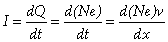(1)

Where e represents the unit charge of the electron and N represents the number of electrons in one Ampere. Substituting the resulting value of I in the scalar version of the Biot-Savart equation then allowed doing away with the time element in this equation also: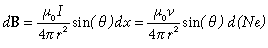(2)

Without going into the detail of his derivation, which is very clearly laid out in his paper (, Equations (1) to (26)), let us only mention that the final stage of this development consists in spherically integrating the electron magnetic energy, whose density is mathematically deemed to vary from a minimum limit corresponding to re to a maximum limit located at infinity.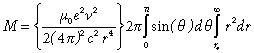(3)

The electron classical radius re is the mandatory lower limit in such an integration to infinity, due to the simple fact that integrating any closer to r = 0 would accumulate more energy than experimental data warrants. After integrating, he obtained: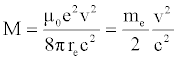(4)

which very precisely corresponds to the total mass of the magnetic field of an electron moving at velocity v. He discovered by the same token that any instantaneous "magnetic mass" increase of an electron is a direct function of the square of its instantaneous velocity.

When this velocity is small with respect to the speed of light, the following classical equation is obtained, allowing clearly determining the contribution of the magnetic component to the rest mass of the electron: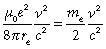(5)

Where re is the classical electron radius (2.817940285E-15 m), and e is the charge of the electron (1.602176462E-19 C), and from which can be concluded that the invariant magnetic component of the electron at rest corresponds to a mass of: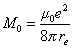(6)

which is exactly half the mass of an electron, the other half being made up of what could be termed its "electric mass", since the electron is an electromagnetic particle. Paying attention to the difference between equations (4) and (6), we observe that M – M0 represents the relativistic mass increment related to instantaneous velocity v. We note also that the translational kinetic energy required to propel the electron at this velocity is absent from the equation. Close analysis and calculation reveals however that the amount of translational kinetic energy required to propel an electron with magnetic mass M at velocity v is exactly equal to the amount of energy captive in the instantaneous relativistic mass increment M – M0.

This means that the total amount of energy that must be communicated to an electron at rest for it to move at any velocity must be defined as an amount of translational kinetic energy plus an equal amount of kinetic energy that momentarily converts to the instantaneous relativistic mass increment related to that velocity.

Etotal = Etranslational + Emagnetic mass increment

Since energy in motion cannot be dissociated from electromagnetism, it can be surmised that an electric component is de facto involved in relation with the half of the energy that in context clearly is “magnetic” in nature, and the only way it can be introduced in context is for this magnetic energy to alternate between this magnetic state and an electric state at the frequency that can be associated to this amount of energy.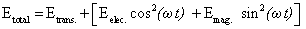(8)

This form in turn immediately suggests the following LC relation to represent the internal structure of the carrying energy of an electron in motion: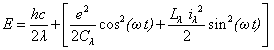(9)

where λ is the wavelength associated to this amount of electromagnetic energy in motion and where the following are the classical equations for calculating capacitance and inductance during a LC cycle: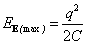and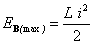(10)

Equation (9) reveals that all probabilities are that the velocity of light of an isolated electromagnetic photon would be maintained because the translational half of its kinetic energy serves to propel at this velocity an equal amount of kinetic energy while the latter permanently oscillates between an electric state and a magnetic state at the frequency determined by the total amount of kinetic energy involved. This structure will be analyzed in detail further on.

The Neglected Classical Maxwellian Space Geometry

Maxwell’s theory is traditionally considered from the mathematical viewpoint offered by his famous equations and understood within the restrictive perspective of plane wave treatment, leaving the space geometry that underlies it to be mostly taken for granted, since it is sufficient for the needs of the continuous wave concept, which in turn is sufficient for precise calculations at the general level. This classical space geometry is of course the traditional Euclidian 3-dimensional flat space geometry to which the time dimension is added to justify motion. Just like the habit of using the electromagnetic tensor to represent a single "electromagnetic field" concept keeps away from immediate attention that both electric and magnetic fields are of equal and separate importance in Maxwell's theory, with different and irreconcilable characteristics, the habit of using plane wave treatment leaves in the background the fact that the wave front of the electromagnetic wave of Maxwell’s theory could only be in spherical expansion from some point-like source, a point-like source which is confirmed out of any doubt by experimental reality for any electromagnetic quanta emission, even if Maxwell's continuous waves had been proven to really exist. Maxwell's theory is in fact the natural end result of the integration of many discoveries made previously. His first equation is In Gauss' law for electricity; his second equation is derived from Faraday's law, his third from Gauss's law on magnetism and his forth is a generalization of Ampere's law. What Maxwell did in fact was unify into one coherent integrated theory all these experimentally confirmed laws that were not clearly linked to each other previously.

But his really brilliant personal contribution was his success in mathematically linking Faraday's law and his modified Ampere's law in such a way that no doubt could remain that light was intimately linked to electricity and magnetism, as confirmed by Faraday’s experiments on light polarization by magnetic fields. Linking them provided as a side benefit the only way ever devised to calculate light velocity from first principles, a velocity that is the only velocity possible from these equations since it rests on the products of only two other fundamental constants, that is, the electric permittivity and magnetic permeability constants of vacuum. As already mentioned, a fundamental and thoroughly verified aspect of Maxwell’s theory is the mandatory state of orthogonality that must exist between the electric and magnetic fields of free moving electromagnetic energy, both fields also being normal to the phase velocity vector that identifies the direction of motion of any point considered on the wave front of the spherically propagating "wave". Experimental reality reveals that this triple orthogonality also applies to the motion of charged massive particles, such as electrons being forced to move in straight line when subjected to equal density external electric and magnetic fields. Indeed, any elementary textbook on electricity and magnetism explains how the vectorial cross product of equal intensity electric and magnetic fields being applied to a charged particle will generate a velocity vector in straight line forcing the particle to move in a direction perpendicular to both resulting forces. The more intense the fields, the faster the particle will move, and whose varying velocity is given in classical electrodynamics from the Lorentz equation, by this well known relation: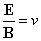(11)

Which resolves to the fixed speed of light “c” for photons, from Maxwell's 4th equation: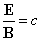(12)

Or rather, in the present context, under the form of a vectorial cross product: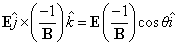(13)

and since angle θ must be equal to 90o by definition in the case of the straight line motion that we are considering: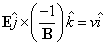(14)

where v is the velocity vector.

The orthogonal bases shown in Figure 3 will be used in this paper:

a) 3D rectangular x-y-z coordinate system, and corresponding rectangular unit vectors base and

b) The correspondingly oriented rectangular electromagnetic fields vs velocity vector base:

It is generally understood also that despite the precision of the calculations that Maxwell's theory allows for electromagnetic energy, his theory is deemed unable to directly describe photons as discrete localized moving electromagnetic particles since it is grounded on the notion that electromagnetic energy is a continuous wave phenomenon.

Discrete Particles as the Only Possible Support of Electromagnetic Properties

So, whatever the final solution will be, it will mandatorily involve this all pervading underlying medium in what we consider as the total vacuum of space.

The Issue of Intensity Conservation with Maxwell's Spherically Expanding Wave Concept

This leads to attempt clarifying why an acceptable description of electromagnetic photons as stable permanently localized moving particles, in line with their demonstrated point-like localization at the moments of emission and capture, more than one century ago, has not yet been successfully reconciled with the verified aspects of Maxwell's theory, particularly after Louis de Broglie elaborated his intriguingly promising hypothesis . According to Maxwell's theory, the electric and magnetic aspects of an electromagnetic wave must by necessity always be in phase at the wave front (Figure 4), that is, at maximum at the same moment, for the wave to exist at all and propagate.When both aspects are 90o out of phase, we obtain a standing wave (Figure 5). But as an intriguing dead end in Maxwell's theory, when both aspects are set 180o out of phase, we end up with the exact equivalent of both aspects being in phase (Figure 4), But we will see further on that far from beingto a plane surface at the infinitesimal level which is the origin of the "plane wave" equations set. But space being three-dimensional, such treatment with the plane wave analogy can only be a mathematical approximation obscuring the fact that if such an electromagnetic wave really physically existed, it could only be in spherical expansion from its initial point-like state, assuming unbounded isotropic expansion. So plane wave treatment applied to Maxwell's theory currently does not describe electromagnetic energy as it starts existing at its pointlike source, but only after the expanding wave has begun to propagate. Also, the geometry of such a spherically propagating wave would be much more similar to the spherical expansion of a sound wave from its point-like source in some underlying medium than to the propagation of waves on a plane liquid surface that immediately comes to mind when thinking about plane wave treatment. It then becomes very difficult to accept the idea that the initial intensity of the point source of the wave could be arbitrarily multiplied in such a way that it could be measured as equal to the energy of the source at any point of the expanding spherical wave front at any arbitrary distance from the punctual source, as plane wave treatment seems to allow. So the habit of dealing with the state of orthogonality of both fields with respect to each other and to the direction of motion in space of any point on the already expanding wave front always leaves in the background the fact that such a spherically expanding wave can only be a single electromagnetic event originating from a single point-like source. a dead end in physical reality, this 180o dephasing will turn out to be in perfect harmony with the LC oscillation for which we will give the mathematic development (Figure 8). Also, it is the conjunction of both fields, in phase and at right angle with each other at all points of the wave front that is deemed to maintain the intensity of the energy of the wave at every points of the wave front, despite the inherent spherical spread involved from the mandatory point-like origin of such a wave, if it really existed. This issue is of course familiar to all but is apparently seen as an unavoidable axiom, no doubt resting on the comfortable fact that plane wave treatment allows precise calculation anyway. Mathematically speaking, when any point of the curved spherical wave front surface is considered, this surface can be locally approximated

Figure 4: Electric and magnetic fields in phase, or 180o out of phase, in classical electromagnetism.

Applying Electromagnetic Properties to Maxwell's Spherically Expanding Wave’s Point-like Initial State

Now, considering that such an electromagnetic event is a single event, could it not be imagined that after appearing at its point-like origin, it could be represented as remaining locally point-like as it starts moving, harmonically oscillating as it moves, which is what de Broglie's hypothesis implies, instead of spherically expanding as Maxwell's theory implies by definition?

This would involve a precise trajectory being followed by this electromagnetic event, which would then behave point-like from emission to capture, which would in turn be in total harmony with the verified f

Select your language of interest to view the total content in your interested language

Article Usage

• Total views: 10263
• [From(publication date):
March-2016 - Oct 22, 2019]
• Breakdown by view type
• HTML page views : 9928Can't read the image? click here to refresh